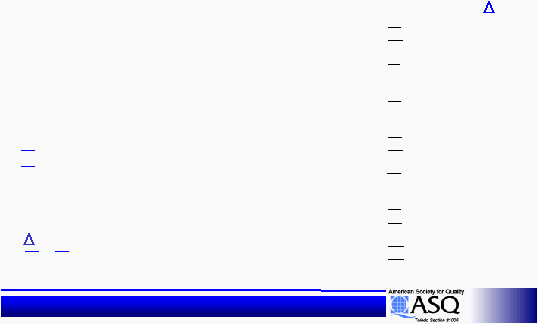< > unit5ReadOnly - page 10 of 13 Six Sigma Six Sigma Training - 6 SigmaStatistical Design of Experiments
Statistical Design of Experiments
A1 =
A2 =
B1 =
B2 =
C1 =
C2 =
D1 =
D2 =
E1 =
E2 =
F1 =
F2 =
G1 =
G2 =
A1
=
A2 =
B1
=
B2 =
C1
=
C2 =
D1
=
D2 =
E1
=
E2 =
F1
=
F2 =
G1
=
G2 =
Oil Consumption (oz/hr)
Oil Consumption (oz/hr)
Level Sums
Level Averages
7.6
9.6
8.1
9.1
8.1
9.1
8.3
8.9
8.3
8.9
12.6
4.6
8.2
9.0
1.9
2.4
2.0
2.3
2.0
2.3
2.1
2.2
2.1
2.2
3.2
1.2
2.1
2.3
0.50
0.25
0.25
0.15
0.15
2.00
0.20
Example
Level Sums
A1 = 2.4 + 0.9 + 1.1 + 3.2 = 7.6
A2 = 3.5 + 1.3 + 1.3 + 3.5 = 9.6
Example
Level Averages
A1 = 7.6 / 4 =
1.9
A2 =
9.6 / 4 = 2.4
Example
A1 -
A2 =
|1.9 -
2.4|
=
0.50